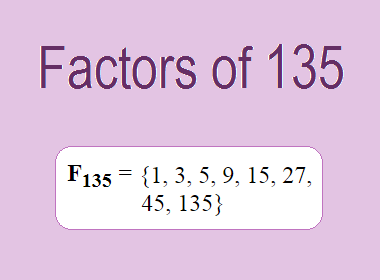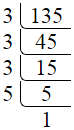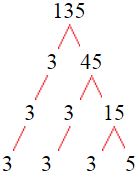# Factors of 135The factors of 135 are 1, 3, 5, 9, 15, 27, 45, and 135 i.e. F135 = {1, 3, 5, 9, 15, 27, 45, 135}. The factors of 135 are all the numbers that can divide 135 without leaving a remainder.

We can check if these numbers are factors of 135 by dividing 135 by each of them. If the result is a whole number, then the number is a factor of 135. Let's do this for each of the numbers listed above:

·        1 is a factor of 135 because 135 divided by 1 is 135.

·        3 is a factor of 135 because 135 divided by 3 is 45.

·        5 is a factor of 135 because 135 divided by 5 is 27.

·        9 is a factor of 135 because 135 divided by 9 is 15.

·        15 is a factor of 135 because 135 divided by 15 is 9.

·        27 is a factor of 135 because 135 divided by 27 is 5.

·        45 is a factor of 135 because 135 divided by 45 is 3.

·        135 is a factor of 135 because 135 divided by 135 is 1.

## How to Find Factors of 135?

1 and the number itself are the factors of every number. So, 1 and 135 are two factors of 135. To find the other factors of 135, we can start by dividing 135 by the numbers between 1 and 135. If we divide 135 by 2, we get a remainder of 1. Therefore, 2 is not a factor of 135. If we divide 135 by 3, we get a remainder of 0. Therefore, 3 is a factor of 135.

Next, we can check if 4 is a factor of 135. If we divide 135 by 4, we get a remainder of 3. Therefore, 4 is not a factor of 135. We can continue this process for all the possible factors of 135.

Through this process, we can find that the factors of 135 are 1, 3, 5, 9, 15, 27, 45, and 135. These are the only numbers that can divide 135 without leaving a remainder.

********************

********************

## Properties of the Factors of 135

The factors of 135 have some interesting properties. One of the properties is that the sum of the factors of 135 is equal to 240. We can see this by adding all the factors of 135 together:

1 + 3 + 5 + 9 + 15 + 27 + 45 + 135 = 240

Another property of the factors of 135 is that they are all odd numbers. This is because 135 is an odd number, and any even number cannot divide an odd number without leaving a remainder.

Another property of the factors of 135 is that the prime factors of 135 are 3 and 5 only.

## Applications of the Factors of 135

The factors of 135 have several applications in mathematics. One of the applications is in finding the highest common factor (HCF) of two or more numbers. The HCF is the largest factor that two or more numbers have in common. For example, to find the HCF of 135 and 81, we need to find the factors of both numbers and identify the largest factor they have in common. The factors of 135 are 1, 3, 5, 9, 15, 27, 45, and 135. The factors of 81 are 1, 3, 9, 27, and 81. The largest factor that they have in common is 27. Therefore, the HCF of 135 and 81 is 27.

Another application of the factors of 135 is in prime factorization. Prime factorization is the process of expressing a number as the product of its prime factors. The prime factors of 135 are 3 and 5, since these are the only prime numbers that can divide 135 without leaving a remainder. Therefore, we can express 135 as:

135 = 3 × 3 × 3 × 5

We can do prime factorization by division and factor tree method also. Here is the prime factorization of 135 by division method,135 = 3 × 3 × 3 × 5

Here is the prime factorization of 135 by the factor tree method,135 = 3 × 3 × 3 × 5

## Conclusion

The factors of 135 are the numbers that can divide 135 without leaving a remainder. The factors of 135 are 1, 3, 5, 9, 15, 27, 45, and 135. The factors of 135 have some interesting properties, such as being odd numbers and having a sum of 240. The factors of 135 have several applications in mathematics, such as finding the highest common factor and prime factorization.